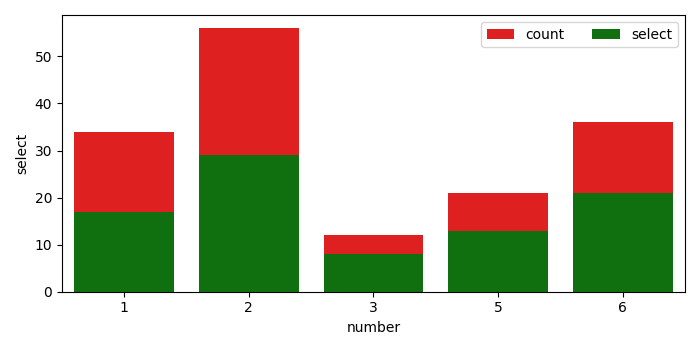# How to create a stacked bar chart for my DataFrame using Seaborn in Matplotlib?

To create a stacked bar chart, we can use Seaborn's barplot() method, i.e., show point estimates and confidence intervals with bars.

• Create df using Pandas Data Frame.

• Using barplot() method, create bar_plot1 and bar_plot2 with color as red and green, and label as count and select.

• To enable legend, use legend() method, at the upper-right location.

• To display the figuree, use show() method.

## Example

import pandas
import matplotlib.pylab as plt
import seaborn as sns
plt.rcParams["figure.figsize"] = [7.00, 3.50]
plt.rcParams["figure.autolayout"] = True
df = pandas.DataFrame(dict(
number=[2, 5, 1, 6, 3],
count=[56, 21, 34, 36, 12],
select=[29, 13, 17, 21, 8]
))
bar_plot1 = sns.barplot(x='number', y='count', data=df, label="count", color="red")
bar_plot2 = sns.barplot(x='number', y='select', data=df, label="select", color="green")
plt.legend(ncol=2, loc="upper right", frameon=True)
plt.show()

## Output